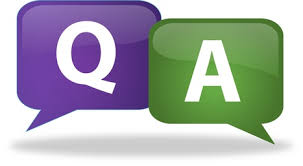# SCC Education

## Rational Number test paperRational Number

1. Write:
(i) The rational number that does not have a reciprocal.
(ii) The rational numbers that is equal to their reciprocals.
(iii) The rational number that is equal to its negative.
(iv) The additive inverse of a negative number

2. Fill in the blanks.
(i) Zero has __________ reciprocal.
(ii) The numbers __________ and __________ are their own raciprocals
(iii) The reciprocal of − 5 is __________.
(v) The product of two rational numbers is always a __________.
(vi) The reciprocal of a positive rational number is __________.

(vii)The number which can be written in the form of p/q , where q≠0, is called _______ number.
(A) Rational (B) Irrational (C) Real (D) Natural

(viii) All rational numbers have multiplicative inverse except _______ .
(A) -1 (B) 1 (C) 0 (D) None

(ix)The sum of any two rational numbers is a ___________ number.
(A) Even (B) Real (C) Rational (D) Natural

(x). 1 A rational number p/q is said to be in the simplest form if the HCF of p and q is
(a) 2 (b) 1 (c) 0 (d) 3

(xi) Between any two distinct rational numbers there exist
(a) Finite rational numbers (b) Infinite rational numbers (a) No rational number (d) none of the above

(xii) A rational number a/b is greater than c/d if

(xiii) .4 Is zero a rational number
(a) Yes (b) No (c) Can’t say

(xiv) Rational numbers are not closed under
(a) Addition (b) Multiplication (c) Division (d) Subtraction

(xv) If the additive inverse of “b” is “a” then:

(A) ab=1 (B) a=b (C) a+b=0 (D) a-b=0 3.

Solve:

1. If you subtract 1/2 from a number and multiply the result by 1/2, you get 1/8. What is the number?

2. Three consecutive integers are such that when they are taken in increasing order and multiplied by 2, 3, and 4 respectively, they add up to 74. Find these numbers.

4. Represent the following rational numbers on the number line (a) -1/4 (b) -11/5 (c) -38/5 (d) -7/10 (e) -5/3

5. Find two rational numbers between (i) -2 and 2. (ii) -1 and 0. 6. Insert six rational numbers between (i) -1/3 and -2/3 (ii) ¼ and ½

circle-theorem-2
circle-theorem-1
Theorems-of-triangles
important-rule-for-circle
surface-area-and-volume
herons-formula
circle
Area-of-parallelograms
elementry-algebra-questions
polynomials
formula-sheet-maths
co ordinate geometry

mensuration